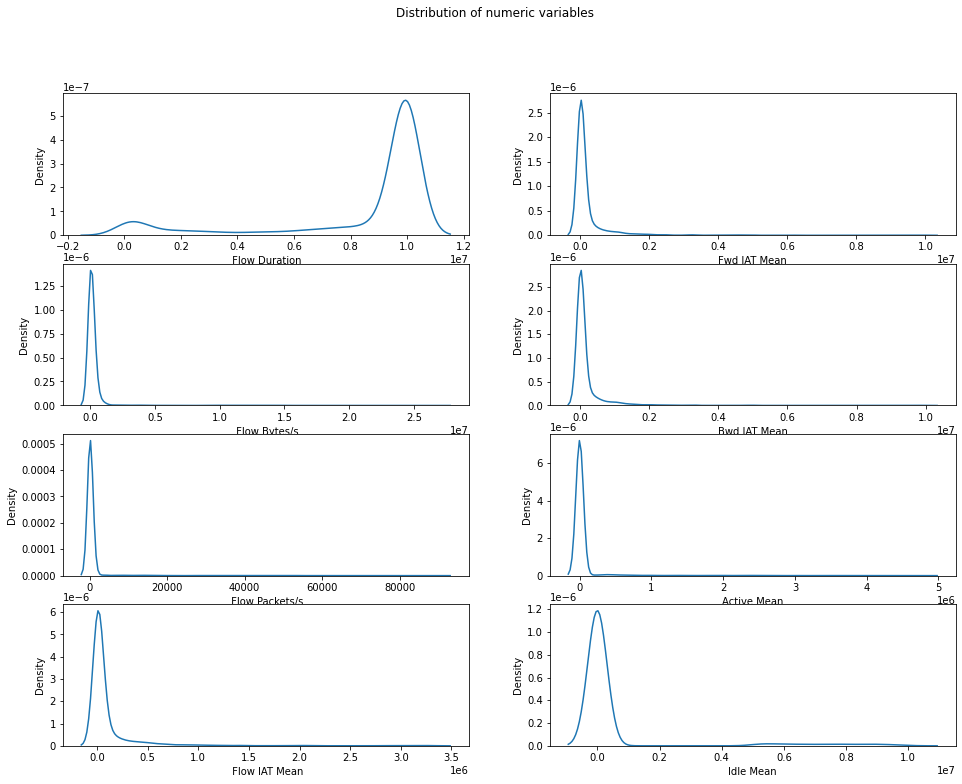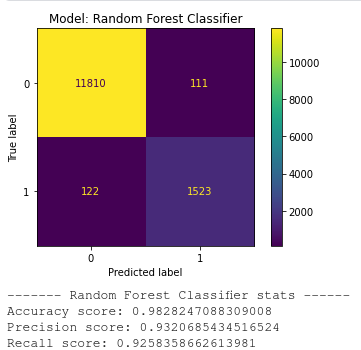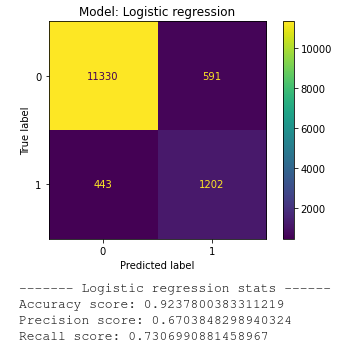# Understanding the data

• FIAT: Forward Interval Arrival Time, he time between two packets sent forward direction (mean, min, max, std).
• BIAT: Backward Inter Arrival Time, the time between two packets sent backwards (mean, min, max, std).
• Flow IAT: Flow Inter Arrival Time, the time between two packets sent in either direction (mean, min, max, std).
• Active: The amount of time time a flow was active before going idle (mean, min, max, std).
• Idle: The amount of time time a flow was idle before becoming active (mean, min, max, std).
• Flow Bytes/s: Flow Bytes per second.
• Flow Packets/s: Flow Packets per second.
• Duration: the duration of the flow.

## Scenario B: characterizing the usage

• Audio traffic was captured from any continuous stream of data from Spotify.
• Browsing is any HTTP and HTTPS traffic generated by users while on Chrome or Firefox.
• Chatting identifies instant-messaging apps, such as Facebook, Skype, ICQ, etc.
• File-transfer identifies traffic that occurred through SFTP, FTPS and Skype file transfers.
• Mail identifies traffic that, obviously, delivered or received mail through SMTP/S, POP3/SSL and IMAP/SSL.
• P2P is used to share file-sharing protocols like torrenting.
• Video traffic was captured from any continuous stream of data from YouTube and Vimeo using Chrome and Firefox.
• VoIP groups all traffic generated by voice applications, such as Facebook, Hangouts and Skype.Distribution plots for each of the features

# Scenario A: Classifying between TOR and non-TOR traffic

`import pandas as pdimport numpy as npimport matplotlib.pyplot as pltimport seaborn as snsfrom sklearn.ensemble import RandomForestClassifierfrom sklearn.linear_model import LogisticRegressionfrom sklearn.model_selection import train_test_splitfrom sklearn.metrics import confusion_matrix, accuracy_score, precision_score, recall_score, ConfusionMatrixDisplayfrom sklearn.preprocessing import StandardScaler, OneHotEncoderfrom sklearn.pipeline import Pipelinefrom sklearn.compose import ColumnTransformerfrom sklearn import set_config`
`processor_1 = ('OneHotEncoder', OneHotEncoder(), [' Protocol'])processor_2 = ('StdScaler', StandardScaler(), [' Flow Duration', ' Flow Bytes/s', ' Flow Packets/s', ' Flow IAT Mean', 'Fwd IAT Mean', 'Bwd IAT Mean', 'Active Mean', 'Idle Mean'])preprocessor = ColumnTransformer( [processor_1, processor_2] )`
`def quickFit(modelName, model, X_train, X_test, y_train, y_test):    """Fits a model to a given dataset and displays accuracy, precision score and recall score.                                 The function supposes that a preprocessor has already been created."""    global preprocessor    model = Pipeline(steps=[('Preprocessing', preprocessor), (modelName, model)])    model.fit(X_train, y_train)    y_pred = model.predict(X_test)        print('\n----- ' + modelName + ' -----')    print(confusion_matrix(y_test, y_pred))    print('Accuracy score: ' + str(accuracy_score(y_test, y_pred)))    print('Precision score: ' + str(precision_score(y_test, y_pred)))    print('Recall score: ' + str(recall_score(y_test, y_pred)))`

## Generating our modelsRandom Forest Classifier confusion matrix and stats.Logistic regression confusion matrix and stats.

# Scenario B: Characterizing usage

`def quickFit(modelName, model, X, y):    global preprocessor        X_train, X_test, y_train, y_test = train_test_split(X, y, test_size=0.2, random_state=0)    le = LabelEncoder()    le.fit_transform(y_train)    le.transform(y_test)    model = Pipeline(steps=[('Preprocessor', preprocessor), (modelName, model)])    model.fit(X_train, y_train)    y_pred = model.predict(X_test)    print("\n-----" + modelName + ' -----')    print(confusion_matrix(y_test, y_pred))    print(classification_report(y_test, y_pred))`

--

--